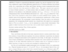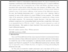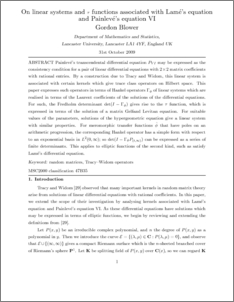# On linear systems and τ functions associated with Lamé's equation and Painlevé's equation VI.

Blower, Gordon (2011) On linear systems and τ functions associated with Lamé's equation and Painlevé's equation VI. Journal of Mathematical Analysis and Applications, 376 (1). pp. 294-316. ISSN 0022-247XPreview
PDF (Painleve.pdf)
Painleve.pdfPreview
PDF (Painleve.pdf)
Painleve.pdf

## Abstract

Painleve's transcendental differential equation PVI may be expressed as the consistency condition for a pair of linear differential equations with 2 by 2 matrix coefficients with rational entries. This linear system is assocaited with certain kernels which give trace class operatos on Hilbert space. This paper expresses such operators in terms of Hankel operators \Gamma_\phi of linear systems. For such, the Fredholm determinant \det (I-\Gamma_\phi P_(t, \infty )) gives rise to the tau function, which is shown to be the solution of a matrix Gelfand--Levitan equation. For meromorphic transfer functions that have poles on an arithmetric progression, the corresponding Hankel operator has a simple form with respect to an exponential basis in L^2(0, \infty ); so \det (I-\Gamma_\phi)can be expressed as a series of finite determinants. This applies to ellliptic functios of the second kind, such as satisfy Lame's differential equation.

Item Type:
Journal Article
Journal or Publication Title:
Journal of Mathematical Analysis and Applications
Uncontrolled Keywords:
/dk/atira/pure/researchoutput/libraryofcongress/qa
Subjects:
Departments:
ID Code:
28028
Deposited By:
Deposited On:
02 Nov 2009 11:35
Refereed?:
Yes
Published?:
Published